SSC CGL Previous Year Questions: Simplification and Square & Cube Root- 1

# SSC CGL Previous Year Questions: Simplification and Square & Cube Root- 1 - SSC CGL

Test Description

## 20 Questions MCQ Test SSC CGL (Tier - 1) - Previous Year Papers (Topic Wise) - SSC CGL Previous Year Questions: Simplification and Square & Cube Root- 1

SSC CGL Previous Year Questions: Simplification and Square & Cube Root- 1 for SSC CGL 2023 is part of SSC CGL (Tier - 1) - Previous Year Papers (Topic Wise) preparation. The SSC CGL Previous Year Questions: Simplification and Square & Cube Root- 1 questions and answers have been prepared according to the SSC CGL exam syllabus.The SSC CGL Previous Year Questions: Simplification and Square & Cube Root- 1 MCQs are made for SSC CGL 2023 Exam. Find important definitions, questions, notes, meanings, examples, exercises, MCQs and online tests for SSC CGL Previous Year Questions: Simplification and Square & Cube Root- 1 below.
Solutions of SSC CGL Previous Year Questions: Simplification and Square & Cube Root- 1 questions in English are available as part of our SSC CGL (Tier - 1) - Previous Year Papers (Topic Wise) for SSC CGL & SSC CGL Previous Year Questions: Simplification and Square & Cube Root- 1 solutions in Hindi for SSC CGL (Tier - 1) - Previous Year Papers (Topic Wise) course. Download more important topics, notes, lectures and mock test series for SSC CGL Exam by signing up for free. Attempt SSC CGL Previous Year Questions: Simplification and Square & Cube Root- 1 | 20 questions in 20 minutes | Mock test for SSC CGL preparation | Free important questions MCQ to study SSC CGL (Tier - 1) - Previous Year Papers (Topic Wise) for SSC CGL Exam | Download free PDF with solutions
 1 Crore+ students have signed up on EduRev. Have you?
SSC CGL Previous Year Questions: Simplification and Square & Cube Root- 1 - Question 1

### What is the value of: 2 of 3 ÷ 3 × 2 + {4 × 3 – (5 × 2 + 3)} = ?       (SSC MTS 2018)

Detailed Solution for SSC CGL Previous Year Questions: Simplification and Square & Cube Root- 1 - Question 1

2 of 3 ÷ 3 × 2 + {4 × 3 – (5 × 2 + 3)}
⇒ 2 × 1 × 2 + {12 – (13)}
⇒ 2 × 2 + {– 1}
⇒ 4 – 1
⇒ 3

SSC CGL Previous Year Questions: Simplification and Square & Cube Root- 1 - Question 2

### What is the value of: (9 ÷ 30)2 × 2.4 + 0.3 o f 12 × (1 – 0.3)2 + 9 × (0.3)2 = ?         (SSC MTS 2018)

Detailed Solution for SSC CGL Previous Year Questions: Simplification and Square & Cube Root- 1 - Question 2

(9 ÷ 30)2 × 2.4 + 0.3 of 12 × (1 – 0.3)2 + 9 × (0.3)2 = ?
⇒ (0.3)2 × 2.4 + 3.6 × (0.7)2 + 9 × (0.09)
⇒ (0.09) × 2.4 + 1.764 + 9 × (0.09)
⇒ 1.98 + 0.81
⇒ 2.79

SSC CGL Previous Year Questions: Simplification and Square & Cube Root- 1 - Question 3

### The value of 15.2 + 5.8 ÷ 2.9 × 2 – 3.5 × 2 ÷ 0.5 is equal to:     (SSC CGL-2018)

Detailed Solution for SSC CGL Previous Year Questions: Simplification and Square & Cube Root- 1 - Question 3

15.2 + 5.8 ÷ 2.9 x 2 - 3.5 x 2 ÷ 0.5
15.2 + 2 x 2 - 7 x 2
15.2 + 4 – 14 = 5.2

SSC CGL Previous Year Questions: Simplification and Square & Cube Root- 1 - Question 4

The value of 4.5 – (3.2 ÷ 0.8 × 5) + 3 × 4 ÷ 6 is:       (SSC CGL-2018)

Detailed Solution for SSC CGL Previous Year Questions: Simplification and Square & Cube Root- 1 - Question 4

4.5 – (3.2 ÷ 0.8 x 5) + (3 x 4 ÷ 6)
= 4.5 – (4 x 5) + 3 x (2/3)
= 4.5 – 20 + 2 = –13.5

SSC CGL Previous Year Questions: Simplification and Square & Cube Root- 1 - Question 5

The simplified value of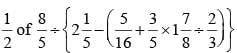is:        (SSC CHSL-2018)

Detailed Solution for SSC CGL Previous Year Questions: Simplification and Square & Cube Root- 1 - Question 5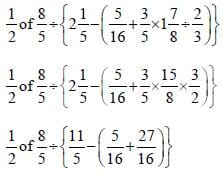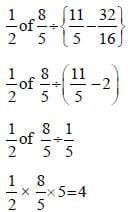SSC CGL Previous Year Questions: Simplification and Square & Cube Root- 1 - Question 6

The simplified value ofis :      (SSC CHSL-2018)

Detailed Solution for SSC CGL Previous Year Questions: Simplification and Square & Cube Root- 1 - Question 6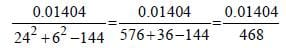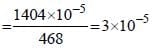SSC CGL Previous Year Questions: Simplification and Square & Cube Root- 1 - Question 7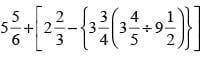is equal To:        (SSC Sub. Ins. 2018 )

Detailed Solution for SSC CGL Previous Year Questions: Simplification and Square & Cube Root- 1 - Question 7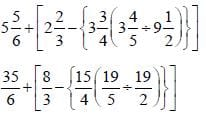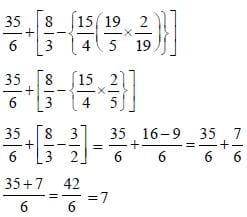SSC CGL Previous Year Questions: Simplification and Square & Cube Root- 1 - Question 8

The value of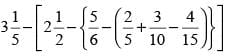is:       (SSC Sub. Ins. 2018 )

Detailed Solution for SSC CGL Previous Year Questions: Simplification and Square & Cube Root- 1 - Question 8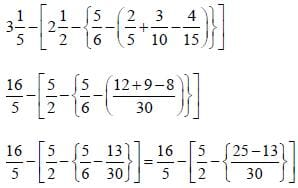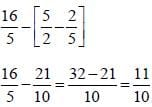SSC CGL Previous Year Questions: Simplification and Square & Cube Root- 1 - Question 9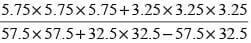is equal to:        (SSC Sub. Ins. 2018 )

Detailed Solution for SSC CGL Previous Year Questions: Simplification and Square & Cube Root- 1 - Question 9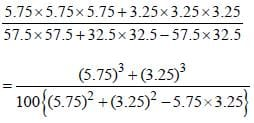This is in the form of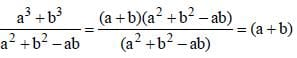Here a = 5.75, b = 3.25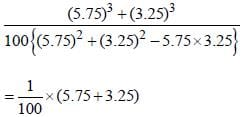= 9/100 = 0.09

SSC CGL Previous Year Questions: Simplification and Square & Cube Root- 1 - Question 10

What is the simplified value of (2 + 1) (22 + 1) (24 + 1) (28 +1)?       (SSC Sub. Ins. 2017)

Detailed Solution for SSC CGL Previous Year Questions: Simplification and Square & Cube Root- 1 - Question 10

According to question.
(2 + 1) (22 + 1) (24 + 1) (28 + 1) = ?
⇒ (2 + 1) (28 + 1) (22+ 1) (24 + 1)
⇒ (29 + 2 + 28 + 1) (26 + 22 + 24 + 1)
⇒ (215 + 214 + 213 + 212 + 211 + 210 + 29 + 28 + 27 + 26 + 25 + 24 + 23 + 22 + 2) + 1
Here, a = 2, n = 15 r = 2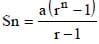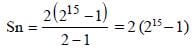⇒ (216 – 2)
∴(216 – 2) + 1 = (216 – 1)
∴ (2 + 1) (22 + 1) (24 + 1) (28 + 1) = (216 – 1)

SSC CGL Previous Year Questions: Simplification and Square & Cube Root- 1 - Question 11

If N =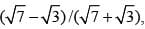then what is the value of N+(1/N)?        (SSC Sub. Ins. 2017)

Detailed Solution for SSC CGL Previous Year Questions: Simplification and Square & Cube Root- 1 - Question 11

Here,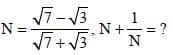Now,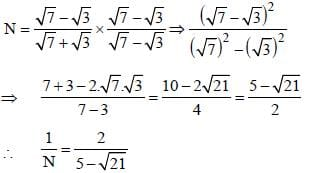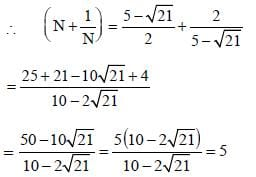SSC CGL Previous Year Questions: Simplification and Square & Cube Root- 1 - Question 12

If 2x – 3(2x – 2) > x – 1 < 2 + 2x, then x can take which of the following values?        (SSC CHSL 2017)

Detailed Solution for SSC CGL Previous Year Questions: Simplification and Square & Cube Root- 1 - Question 12

Here,
2x – 3 (2x – 2) > x – 1 < 2 + 2x
2x – 6x + 6 > x – 1
⇒ 2x – 6x – x > –7
⇒ –5x > –7
x < 7/5 ...(i)
(x – 1) < (2 + 2x)
x – 1 < 2 + 2x
– 3 < x ...(ii)
From (i) and (ii),
x = –2.

SSC CGL Previous Year Questions: Simplification and Square & Cube Root- 1 - Question 13

The sum of two numbers is 15(1/3) and their difference is 4(2/3). The product of the numbers is        (SSC Sub Ins. 2016)

Detailed Solution for SSC CGL Previous Year Questions: Simplification and Square & Cube Root- 1 - Question 13

Let the numbers are x and y.
According to question
x + y = 46/3 ...(i)
x – y = 14/3 ...(ii)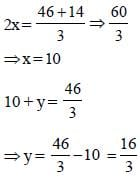Product of number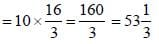SSC CGL Previous Year Questions: Simplification and Square & Cube Root- 1 - Question 14

If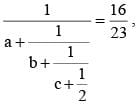then the value of a + b + c       (SSC Sub Ins. 2016)

SSC CGL Previous Year Questions: Simplification and Square & Cube Root- 1 - Question 15

If the numbers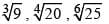are arranged in ascending order, then the right arrangement is  (SSC CGL 2016).

Detailed Solution for SSC CGL Previous Year Questions: Simplification and Square & Cube Root- 1 - Question 15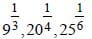L.C.M of Numbers of Powers = 12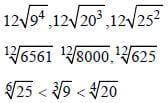"

SSC CGL Previous Year Questions: Simplification and Square & Cube Root- 1 - Question 16

The value of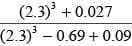is :        (SSC CGL 2016)

Detailed Solution for SSC CGL Previous Year Questions: Simplification and Square & Cube Root- 1 - Question 16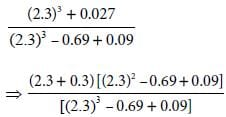⇒ 2.3 + 0.3 = 2.6

SSC CGL Previous Year Questions: Simplification and Square & Cube Root- 1 - Question 17

If 3/4 of a number is 7 more than 1/6 of the number,  then 5/3 ofthe number is :        (SSC CGL 2015)

Detailed Solution for SSC CGL Previous Year Questions: Simplification and Square & Cube Root- 1 - Question 17

Let the number be x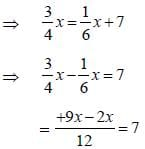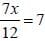∴ x = 12
Now, 5/3 of x = (5/3)x 12 = 20.

SSC CGL Previous Year Questions: Simplification and Square & Cube Root- 1 - Question 18

If x =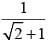then (x + 1) equals to       (SSC CGL 2nd Sit. 2015)

Detailed Solution for SSC CGL Previous Year Questions: Simplification and Square & Cube Root- 1 - Question 18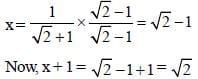SSC CGL Previous Year Questions: Simplification and Square & Cube Root- 1 - Question 19

Choose the incorrect relation(s) from the following:        (SSC CGL 1st Sit. 2015)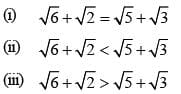Detailed Solution for SSC CGL Previous Year Questions: Simplification and Square & Cube Root- 1 - Question 19

By squaring the given relations, we get (i) and (iii) are incorrect relations from the given statement.

SSC CGL Previous Year Questions: Simplification and Square & Cube Root- 1 - Question 20

The value of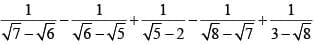is      (SSC CGL 1st Sit. 2015)

Detailed Solution for SSC CGL Previous Year Questions: Simplification and Square & Cube Root- 1 - Question 20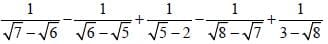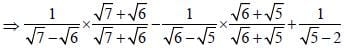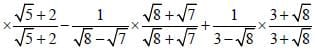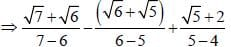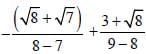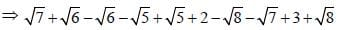⇒ 5

## SSC CGL (Tier - 1) - Previous Year Papers (Topic Wise)

250 tests
Information about SSC CGL Previous Year Questions: Simplification and Square & Cube Root- 1 Page
In this test you can find the Exam questions for SSC CGL Previous Year Questions: Simplification and Square & Cube Root- 1 solved & explained in the simplest way possible. Besides giving Questions and answers for SSC CGL Previous Year Questions: Simplification and Square & Cube Root- 1, EduRev gives you an ample number of Online tests for practice

250 tests